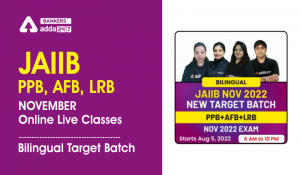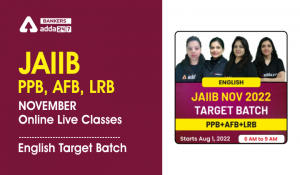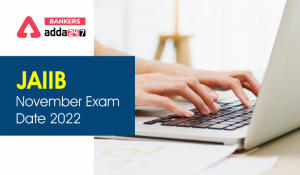Latest Banking jobs   »   Depreciation

# Depreciation Definition, Methods, Factors & Asked Questions in JAIIB Exam

Depreciation: In the JAIIB Exam the topic of Depreciation has much weightage.  When we go through the exam analysis we can notice that a number of questions are seen from Depreciation. From the examination point of view, the aspirants cannot ignore this topic. Each and every concept of Depreciation should be at the tip of a candidate. In the JAIIB Exam, many theoretical questions as well as case studies are asked from the section of Depreciation. So all the JAIIB/CAIIB aspiring candidates must be updated with this topic. In this article, we have provided the handy notes for the topic of Depreciation. This notes will be truly beneficial in the preparation as it will give an overview of Depreciation.

## Depreciation: Definition

A Decrease in the price of fixed assets due to wear and tear, passage of time, and obsolescence is termed as Depreciation. The term Depreciation has been derived from the Latin word “Depritium” which means reduction or devaluation. Assets are the income generating units or those which have a resale value. The assets that are used in business for more than one accounting year are called as Fixed Assets. Depreciation of a machine is included in the operating cost. Mostly the value of assets depreciate but only the price of land and old paintings appreciate.

## Depreciation: Causes

Depreciation is caused due to certain reasons and the main causes are listed below.

• Wear and Tear – After the normal use of any object we can observe wear and tear in them which ultimately leads to depreciation.
• Efflux/Passage of Time– As time passes by the value of an asset gets reduced even if it is not in usage.
• Reduce in the market price– The market price of the asset decreases due to the market conditions or may be there is less demand of that particular asset.
• Obsolescence– An asset is not in use if there is any other invention and when the demand gets permanently changed.

## Need for Depreciation

It is said that depreciation is needed and the main reasons for the need of depreciation is provided below.

• To know the correct profit
• To get the correct financial position
• To make provisions for the replacement of asset

## Factors required for calculation of Depreciation

The data given here are essential for the calculation of Depreciation.

• Actual Price of the Asset
• Approximate Scrap Value and this is calculated when the life of the asset is at end and the asset will be sold.
• The number of years asset will be used approximately

## Depreciation: Methods

Depreciation is calculated by different methods and some of the methods are mentioned here:

• Straight Line Method- In the straight line method depreciation is calculated on the original value of the asset which includes the installation and transportation price but the estimated life of the asset is not considered.

Depreciation= Original Price of the assets- Scrap Value

• Written Down Value Method(WDV) or Declining Balance Method- In the Declining Balance Method Depreciation is charged at a fixed rate on the diminishing balance. In certain cases written down value method is called as Reducing Installment Method.

Depreciation= Last year Value*Percentage Rate

• Sum of the year Digit Method- In this method estimated life of an asset is considered. It is then counted back to 1 and then all the digits are summed up.
• Double Decline Balance Depreciation Method- Double Decline Balance Depreciation Method is same as the Declining Balance Method. In this method fixed percentage rate is double the rate used in straight line method.

Depreciation= Net Book Value*Depreciation Rate

## Depreciation Questions Asked in JAIIB Exam

Directions (1-2): On the basis of the information given below, answer the following questions.
The balance in the accumulated provision for depreciation account of a company as at the beginning of the year 2020-2021 was Rs. 2,00,000 when the original cost of the assets amounted to Rs. 10,00,000. The company charges full 10% depreciation on a straight line basis for all the assets including those which have been either purchased or sold during the year. One such asset costing Rs. 5,00,000 with accumulated depreciation as at the beginning of the year of Rs. 1,00,000 was disposed of during the year.
Q1. Depreciation for the current year is .
(a) Rs. 40,000
(b) Rs. 50,000
(c) Rs. 60,000
(d) Rs. 1,00,000
Ans: (d)
Q2. The balance of the accumulated depreciation account at the end of the year considering the current year’s depreciation charge would be .
(a) Rs. 2,20,000
(b) Rs. 1,50,000
(c) Rs. 1,20,000
(d) Rs. 2,50,00

Ans: (b)

Q3. Deprecation is more by which method during the first and
second year?
(a) Straight line method
(b) Double declining method
(c) Written down method
(d) Sun of the digits method

Ans: (b)

Q4. Depreciation is charged on

(a) Fixed Assets

(b) Current Asset

(c) Capital

(d) Investments

Ans: (a)

Q5. Which of the following statements is incorrect?

(a) Depreciation is a part of the operating cost

(b) Depreciation is a non cash expense

(c) Depreciation is charged on current asset

(d) All are incorrect

Ans: (c)Related Posts:

## FAQs: Depreciation

Q.1 What is meant by Depreciation?

Ans. Decrease in the price of fixed assets because of wear & tear, passage of time and obsolescence is called as Depreciation.

Q.2 What are the main causes of Depreciation?

Ans. The main causes of Depreciation are Wear and Tear, Passage of time, Reduction in the market price and obsolescence.

Q.3 Why do we need Depreciation?

Ans. We need Depreciation to know the correct profit, to show the correct financial position, and to make provisions for replacement of asset.

Q.4 What are the different types of Depreciation?

Ans. The different types of depreciation are Straight Line Method, Declining Balance Method, Double declining balance method.#### Congratulations!Incorrect details? Fill the form again here

•JAIIB PPB, AFB, LRB November Online Live...
•JAIIB PPB, AFB, LRB November 2022 Online...
•JAIIB Result 2022, IIBF JAIIB June Resul...
•Banking Terms in JAIIB PPB, Check Here
•JAIIB November Exam Date 2022 Out, IIBF ...
•Accounting Terms in JAIIB AFB, Check Her...